Quick Introduction

Start

library(dendextend) #

Create a dendrogram (if you don’t know what %>% is, read about it here ) :

dend <- c(1:5) %>% dist %>% hclust("ave") %>% as.dendrogram

Plot:

plot(dend)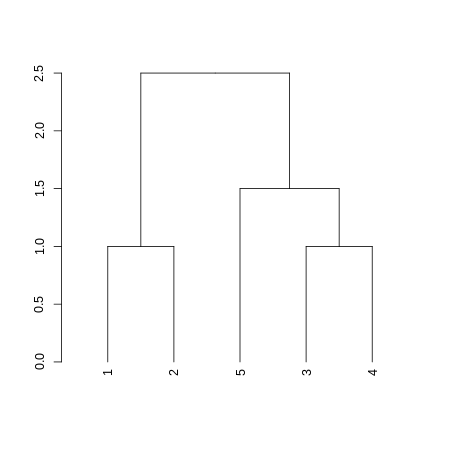Get/set labels

labels(dend)
##  1 2 5 3 4
labels(dend) <- c("A", "B", "extend", "dend", "C")
labels(dend)
##  "A"      "B"      "extend" "dend"   "C"

Get/set labels’ colors

labels_colors(dend)
## NULL
labels_colors(dend) <- rainbow(5)
labels_colors(dend)
##           A           B      extend        dend           C
## "#FF0000FF" "#CCFF00FF" "#00FF66FF" "#0066FFFF" "#CC00FFFF"
plot(dend)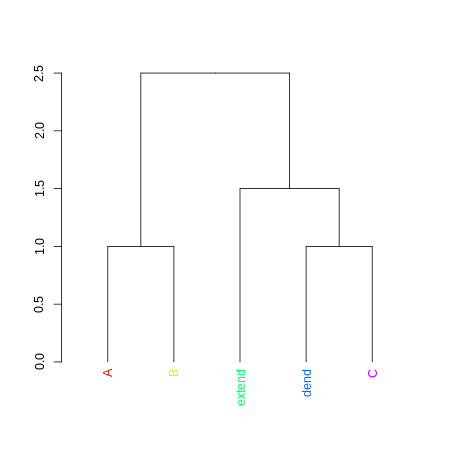Cut-tree, and color branches

cutree(dend, k = 2)
##      A      B   dend      C extend
##      1      1      2      2      2
dend <- color_branches(dend, k = 2)
plot(dend)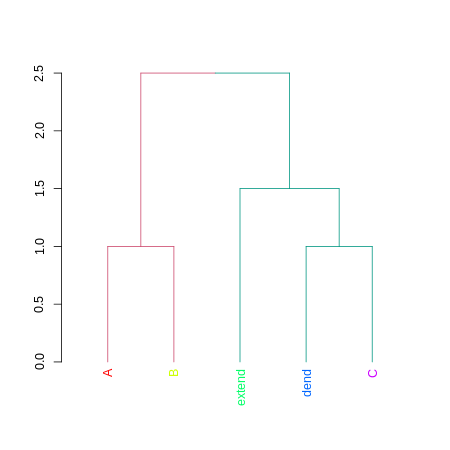Sort

dend2 <- sort(dend)
plot(dend2)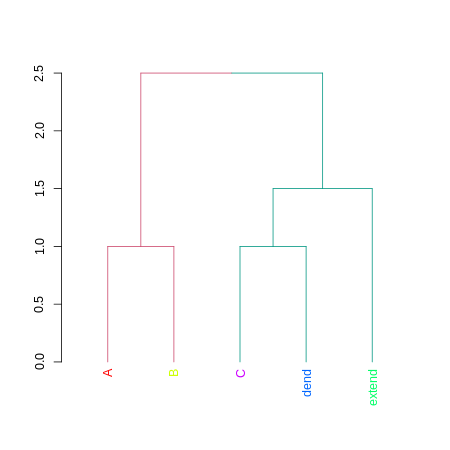Compare

tanglegram( dend,  dend2  )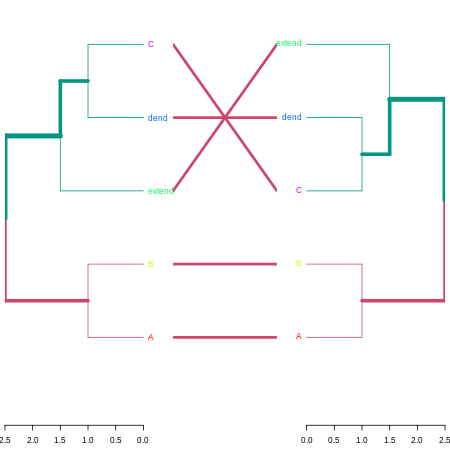Cor

cor_cophenetic( dend,  dend2  )
##  1

ggplot2

library(ggplot2)
ggplot(dend)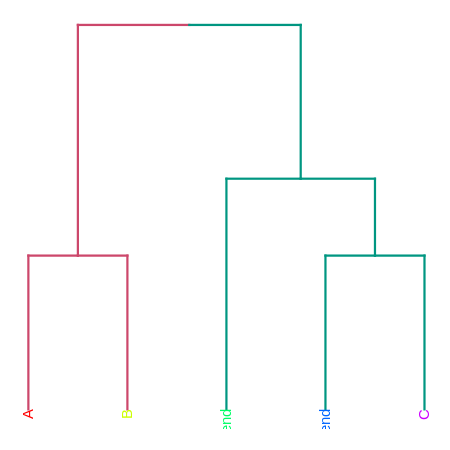Send to plot.ly

# library(plotly)
# set_credentials_file(...)
# you'll need to get it from here: https://plot.ly/ggplot2/getting-started/

# ggplot(dend)
# py <- plotly()
# py\$ggplotly()